# Equations (1) Flashcards Preview

## Pharmacology > Equations (1) > Flashcards

Flashcards in Equations (1) Deck (9):
1

## What is the equation for Volume of Distribution?

### where C = concentration of drug in blood/plasmaAmount of drug in body = Dose administered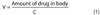2

## What is the equation for Clearance?  What is the unit most commonly used for Clearance?

### where C = concentration of drug in blood/plasmaUnits for Clearance: mL/minClearance is the amount of plasma cleared of the drug per unit time.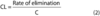3

## What is the equation for Rate of Elimination?

### where CL = Clearance and C = concentration of drug in blood/plasma4

## What is the equation for Half-life?

### where V = volume of distibution and CL = clearance5

## What is the equation for Hepatic Extraction Ratio?

### where ER = extraction ratio, Q = hepatic blood flow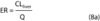6

## Keeping in mind the equation for the rate of Elimination, what is the equation for the Maintenance Dose in an IV infusion?

### where ss = steady state, CL = clearance, TC = target concentration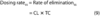7

## If you switched from an IV infusion to an oral dosing regimen, how would you alter the "dosing rate" that you would use in your equations?

### where Dosing Rate = the rate used for IV infusions, F = bioavailability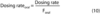8

9